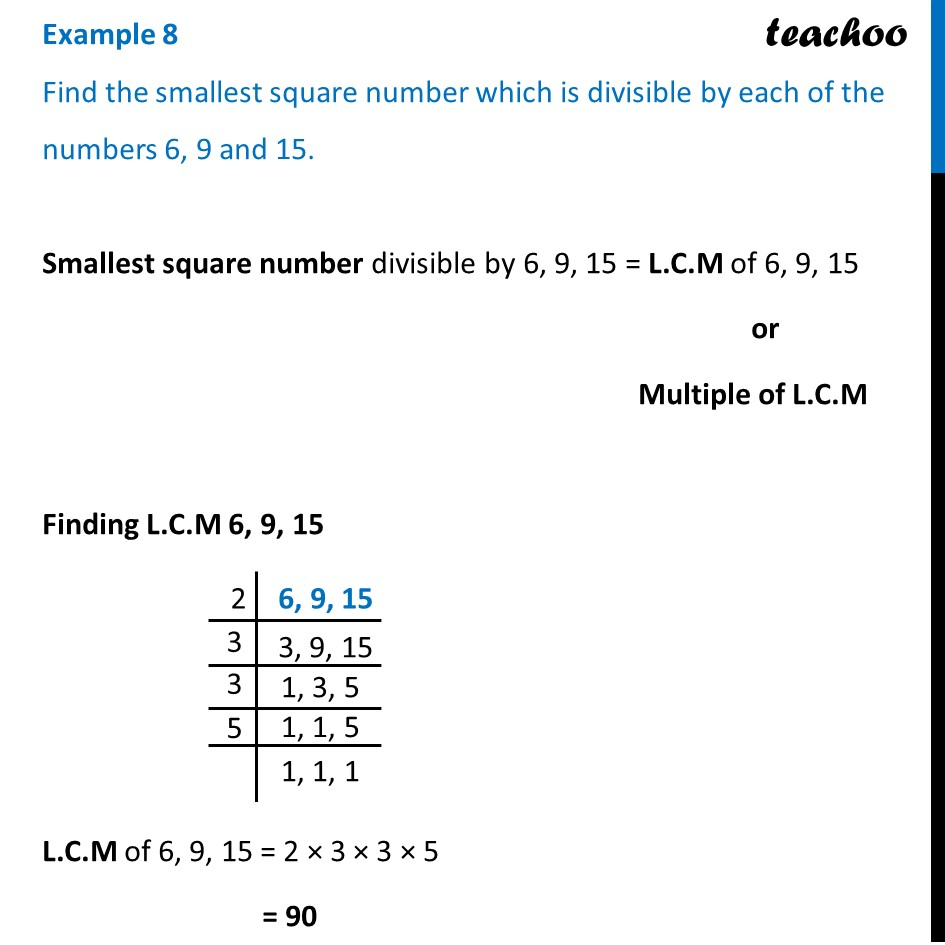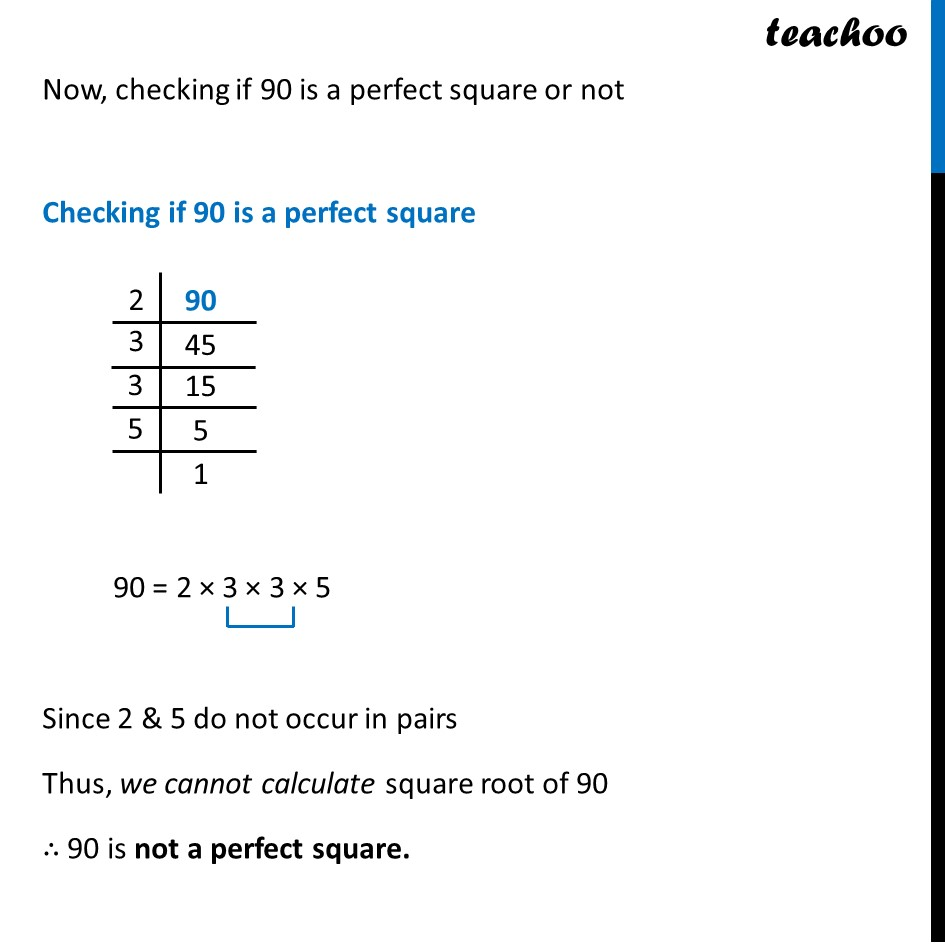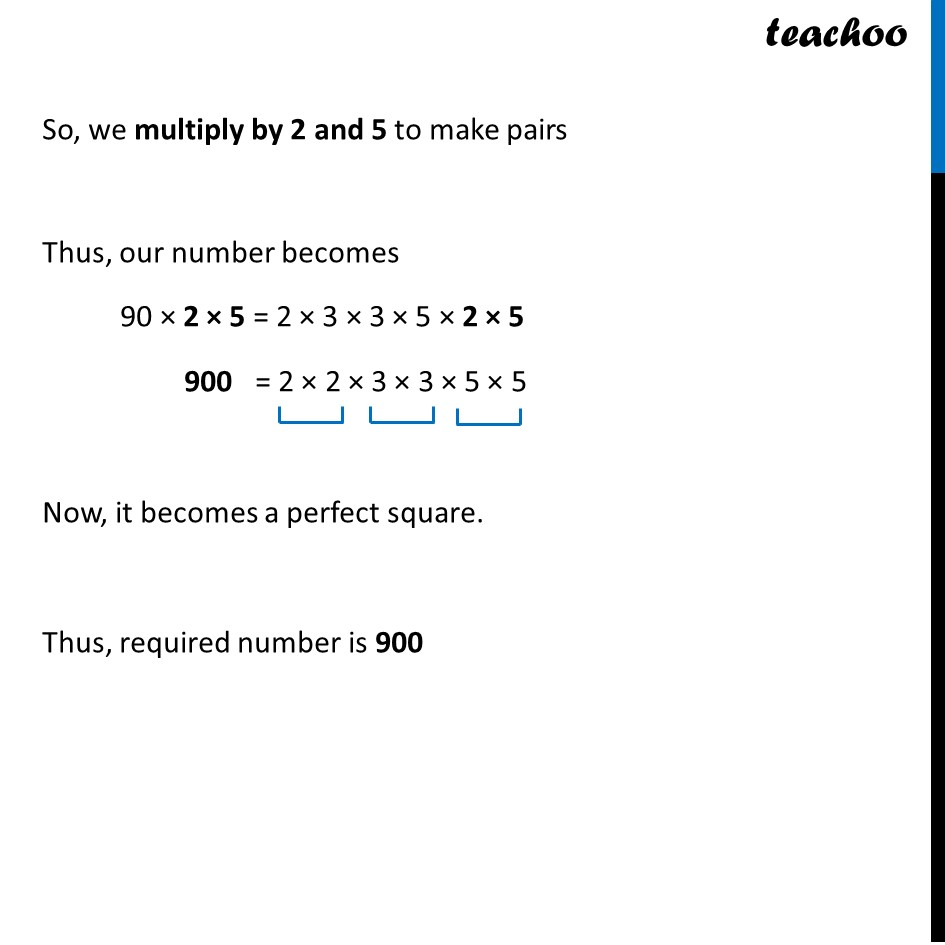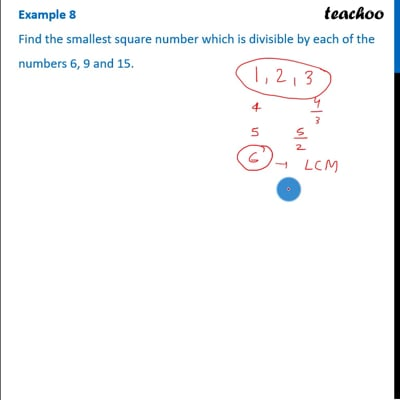Examples

Chapter 6 Class 8 Squares and Square Roots
Serial order wiseThis video is only available for Teachoo black users

Introducing your new favourite teacher - Teachoo Black, at only ₹83 per month

### Transcript

Example 8 Find the smallest square number which is divisible by each of the numbers 6, 9 and 15. Smallest square number divisible by 6, 9, 15 = L.C.M of 6, 9, 15 or Multiple of L.C.M Finding L.C.M 6, 9, 15 L.C.M of 6, 9, 15 = 2 × 3 × 3 × 5 = 90 Now, checking if 90 is a perfect square or not Checking if 90 is a perfect square 90 = 2 × 3 × 3 × 5 Since 2 & 5 do not occur in pairs Thus, we cannot calculate square root of 90 ∴ 90 is not a perfect square. So, we multiply by 2 and 5 to make pairs Thus, our number becomes 90 × 2 × 5 = 2 × 3 × 3 × 5 × 2 × 5 900 = 2 × 2 × 3 × 3 × 5 × 5 Now, it becomes a perfect square. Thus, required number is 900